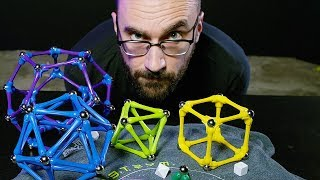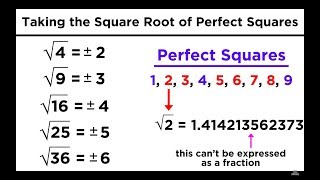○○○ mold ⪢⪢
A sphere and a cube are topologically the same thing since you can just kind of mold one into the other. #math
http://qindex.info/i.php?x=16021Mysterium Cosmographicum
polytope, polygon, polyhedron, platonic solid, tetrahedron, cube, octahedron, dodecahedron, icosahedron
http://qindex.info/i.php?x=342807 Square Roots, Cube Roots, and Other Roots
Square Roots, Cube Roots, and Other Roots
http://qindex.info/i.php?x=4992
----